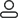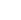LanguageLogin|Sign up
Blog >> Blog Details Page
Understanding of input impedance and output impedance
Posted:03:52 PM November 05, 2018 writer: G

1. Input impedance

The input impedance is the equivalent impedance of a circuit input. Add a voltage source U to the input and measure the current I at the input. The input impedance Rin is U/I. You can think of the input as the end of a resistor. The resistance of this resistor is the input impedance.

The input impedance is no different from a normal reactive component. It reflects the magnitude of the current blocking effect. For a voltage-driven circuit, the higher the input impedance, the lighter the load on the voltage source, and the easier it is to drive. For current-driven circuits, the smaller the input impedance, the lighter the load on the current source. Therefore, we can think that if it is driven by a voltage source, the input impedance is lower. The bigger the better; if it is driven by a current source, the impedance is as small as possible (Note: only suitable for low frequency circuits, in high frequency circuits, also consider the impedance matching problem. Also, if you want to obtain the maximum output power, Consider impedance matching

2. Output impedance

Regardless of the source or amplifier and the power supply, there is a problem with the output impedance. The output impedance is the internal resistance of a signal source. Originally, for an ideal voltage source (including the power supply), the internal resistance should be 0, or the ideal current source. The impedance should be infinite. The output impedance is the most special attention to the circuit design but the actual voltage source can not do this. We often use an ideal voltage source in series with a resistor r to be equivalent to an actual voltage source. The resistor r in series with the ideal voltage source is the internal resistance of the (signal source/amplifier output/power supply). When this voltage source supplies power to the load, a current I flows through the load and is generated on this resistor. I × r voltage drop. This will cause the power supply output voltage to drop, thus limiting the maximum output power (for why the maximum output power is limited, see the next "Impedance Match" question). Similarly, an ideal current Source, output impedance should be infinite, but the actual circuit is impossible

3. Impedance matching

Impedance matching refers to a suitable matching method between signal source or transmission line and load. Impedance matching is divided into low frequency and high frequency.

We start by driving a load from a DC voltage source. Since there is always an internal resistance due to the actual voltage source (please refer to the output impedance), we can use an actual voltage source equivalent to an ideal voltage source. The model of the resistor r series. Assuming the load resistance is R, the power supply potential is U, and the internal resistance is r, then we can calculate the current flowing through the resistor R as: I=U/(R+r), it can be seen that the load The smaller the resistance R is, the larger the output current is. The voltage on the load R is: Uo=IR=U/[1+(r/R)]. It can be seen that the larger the load resistance R, the higher the output voltage Uo. Then calculate the power consumed by the resistor R as:

P=I2×R=[U/(R+r)]2×R=U2×R/(R2+2×R×r+r2)

=U2×R/[(R-r)2+4×R×r]

=U2/{[(R-r)2/R]+4×r}

For a given source, the internal resistance r is fixed, and the load resistance R is chosen by us. Note that [(Rr)2/R], when R=r, [(Rr) 2/R] can obtain the minimum value of 0. At this time, the maximum output power Pmax=U2/(4×r) can be obtained on the load resistor R. That is, when the load resistance is equal to the internal resistance of the signal source, the maximum output power can be obtained by the load. This is one of the impedance matching we often say. For purely resistive circuits, this conclusion is equally applicable to low-frequency circuits and high-frequency circuits. When the AC circuit contains capacitive or inductive impedance, the conclusion is changed, that is, the signal source and The real part of the load impedance is equal, and the imaginary parts are opposite to each other. This is called conjugate matching. In the low frequency circuit, we generally do not consider the matching problem of the transmission line, only consider the situation between the signal source and the load, because of the low frequency signal. The wavelength is very long relative to the transmission line. The transmission line can be regarded as a "short line". The reflection can be ignored (it can be understood as follows: because the line is short, even if it is reflected back, it is the same as the original signal). From the above analysis, we can conclude Conclusion: If we need a large output current, choose a small load R; if If we need a large output voltage, we choose a large load R. If we need the maximum output power, we choose a resistor that matches the internal resistance of the signal source. Sometimes the impedance mismatch has another meaning. For example, some instrument outputs are specific. Under the load conditions, if the load conditions change, it may not reach the original performance, then we will also be called impedance mismatch.

In high-frequency circuits, we must also consider the problem of reflection. When the frequency of the signal is high, the wavelength of the signal is very short. When the wavelength is short compared with the length of the transmission line, the superposition of the reflected signal on the original signal will change. The shape of the original signal. If the characteristic impedance of the transmission line is not equal to the load impedance (ie, it does not match), reflection will occur at the load end. Why does the impedance do not match when it produces reflection and the method of solving the characteristic impedance, involving the second order bias? The solution of differential equations is not elaborated here. For those interested, please refer to the transmission line theory in books on electromagnetic fields and microwaves. The characteristic impedance of transmission lines (also called characteristic impedance) is determined by the structure and material of the transmission line, and The length of the transmission line, as well as the amplitude and frequency of the signal, are independent.

For example, the commonly used CCTV coaxial cable has a characteristic impedance of 75 Ω, while some RF equipment commonly uses a coaxial cable with a characteristic impedance of 50 Ω. Another common transmission line is a flat parallel line with a characteristic impedance of 300 Ω. The TV antenna frame used is more common, used to make the feeder of Yagi antenna. Because the input impedance of the TV's RF input is 75Ω, the 300Ω feeder will not match. How to solve this problem in practice? I don't know. Did you notice that the TV's accessories have a 300Ω to 75Ω impedance converter (a plastic package with a round plug on one end, about two thumbs). Inside is actually a transmission line transformer, which converts the impedance of 300Ω into 75Ω, so that it can be matched. It should be emphasized that the characteristic impedance is not a concept with the resistance we usually understand, it is independent of the length of the transmission line. It cannot be measured by using an ohmmeter. In order not to generate reflection, the load impedance and the characteristic impedance of the transmission line should be equal, which is the resistance of the transmission line. Matching, if the impedance is not matched, what are the adverse consequences? If it does not match, it will form a reflection, energy transfer will not pass, reduce efficiency; will form a standing wave on the transmission line (simple understanding, that is, some places have strong signals, some places The signal is weak), resulting in a decrease in the effective power capacity of the transmission line; the power transmission does not go out, and even the transmitting device may be damaged. If the high-speed signal line on the circuit board does not match the load impedance, oscillation, radiation interference, etc. may occur.

761 1 0 1
• PCB
Prototype
• PCB
Assembly
• SMD
Stencil
 Dimensions: (mm) × Quantity: (pcs) 5 5 10 15 20 25 30 40 50 75 100 120 150 200 250 300 350 400 450 500 600 700 800 900 1000 1500 2000 2500 3000 3500 4000 4500 5000 5500 6000 6500 7000 7500 8000 9000 10000 Other Quantities:(quantity*length*width is greater than 10㎡) OK Layers: 1 2 4 6 8 10 12 Thickness: 0.6 mm 0.8 mm 1.0 mm 1.2 mm 1.6 mm 2.0 mm 2.5 mmQuote now